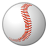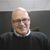# QlikView App Dev

Discussion Board for collaboration related to QlikView App Development.

Announcements
Welcome to Qlik Community! Check out our new navigation! FIND OUT MORE
cancel
Showing results for
Did you mean:Creator II

## subtraction in set analysis

Hi All

In Set Analysis if we use match(LineNo, '1A', '1B', '1C') than all lines value will be added.

Is that possible if I want addition of '1A' & '1B' and subtract '1C' out of these? like '1A' + '1B' - '1C' in single set analysis

Something like this

Sum({\$<LineNo = {'1A', '1B'}-{'1C'}>} AMT)

Thanks

1 Solution

Accepted SolutionsSpecialist

If I understand you have rows where LineNo have values: 1A, 1B and 1C

If you want a set of lines with values 1A and 1B, just {<LineNo={'1A','1B'}>} but if you want to substract the sum of all lines with values 1C, you need to

Sum({<LineNo={'1A','1B'}>} Value) - Sum({<LineNo={'1C'}>} Value)

You can't put this substaction in one line, in the case you are pointing you have the same result in this case:

{<LineNo={'1A','1B'}>} = {<LineNo={'1A','1B'}-{'1C'}>}

11 RepliesCreator II
Author

Match is not needed in Set AnalysisMVP

How can a LineNo be 1A and IC at the same time? What exactly are you trying to do? Can you elaborate a little?Partner - Champion III

What exactly do you mean by add and subtract here? Is it set union and set exclusion you mean or the subtracting and adding values?Creator II
Author

Basically

Sum({\$<LineNo = {'1A', '1B'}>} AMT)  -  Sum({\$<LineNo = {'1C'}>} AMT)

in a Single Set Analysis...something like I mentioned in original post

ThanksCreator II
Author

Basically

Sum({\$<LineNo = {'1A', '1B'}>} AMT)  -  Sum({\$<LineNo = {'1C'}>} AMT)

in a Single Set Analysis...something like I mentioned in original post

ThanksMVP

AFAIK, this isn't possible.Specialist

If I understand you have rows where LineNo have values: 1A, 1B and 1C

If you want a set of lines with values 1A and 1B, just {<LineNo={'1A','1B'}>} but if you want to substract the sum of all lines with values 1C, you need to

Sum({<LineNo={'1A','1B'}>} Value) - Sum({<LineNo={'1C'}>} Value)

You can't put this substaction in one line, in the case you are pointing you have the same result in this case:

{<LineNo={'1A','1B'}>} = {<LineNo={'1A','1B'}-{'1C'}>}Partner - Champion III

No what you show now is something totally different from what you showed in your original post.

The latter is correct. The original post will do a set exclusion of the rows that are satisfied by the set modifiers... not a subtract of the values.MVP

Satish,

Set Analysis is a simple Boolean condition, it returns True or False for every row of data, and that determines whether the specific row is included or excluded from the calculation.

Besides that, Set Analysis cannot perform any mathematical operations - no additions, no subtractions, no divisions and no multiplications. For these purposes, you still need to use multiple aggregation functions with the corresponding mathematical operators between them.

cheers,

Oleg Troyansky

Upgrade your Qlik skills at the Masters Summit for Qlik - coming soon to Milan, Italy and Austin, TX!